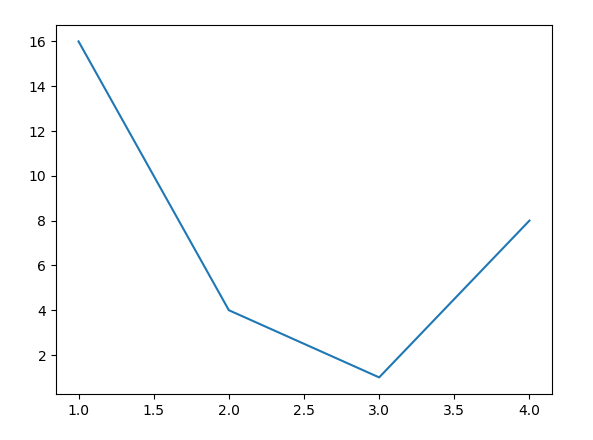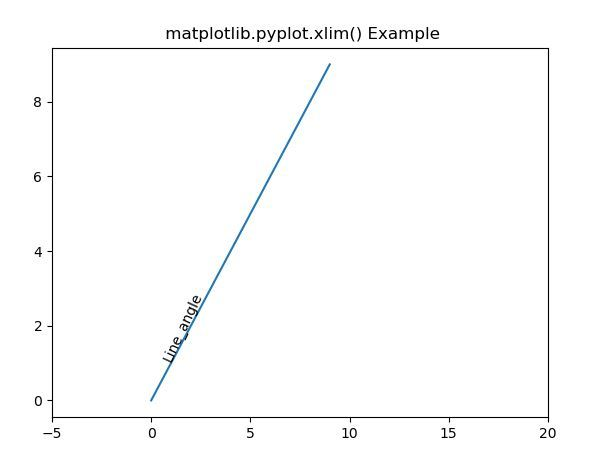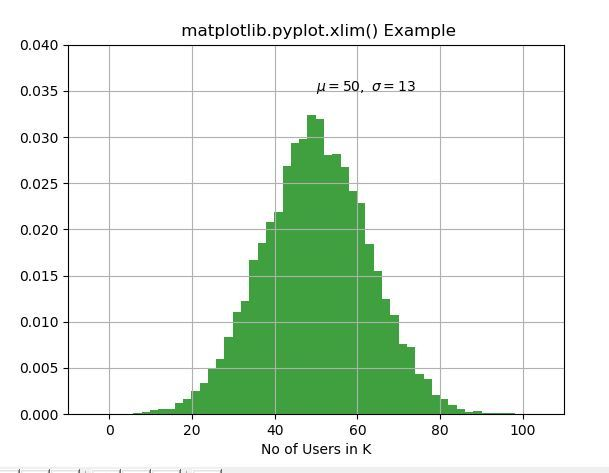# Matplotlib.pyplot.xlim() in Python

Matplotlib is a library in Python and it is numerical – mathematical extension for NumPy library. Pyplot is a state-based interface to a Matplotlib module which provides a MATLAB-like interface. There are various plots which can be used in Pyplot are Line Plot, Contour, Histogram, Scatter, 3D Plot, etc.
#Sample Code

 # sample code  import matplotlib.pyplot as plt        plt.plot([1, 2, 3, 4], [16, 4, 1, 8])   plt.show()

Output:## matplotlib.pyplot.xlim() Function

The xlim() function in pyplot module of matplotlib library is used to get or set the x-limits of the current axes.
Syntax:

matplotlib.pyplot.xlim(*args, **kwargs)

Parameters: This method accept the following parameters that are described below:

• left: This parameter is used to set the xlim to left.
• right: This parameter is used to set the xlim to right.
• **kwargs: This parameter is Text properties that is used to control the appearance of the labels.

Returns: This returns the following:

• left, right: This returns the tuple of the new x-axis limits.

Below examples illustrate the matplotlib.pyplot.ylim() function in matplotlib.pyplot:

Example-1:

 # Implementation of matplotlib function  import matplotlib.pyplot as plt  import numpy as np     h = plt.plot(np.arange(0, 10), np.arange(0, 10))  plt.xlim([-5, 20])  l1 = np.array((1, 1))  angle = 65    th1 = plt.text(l1, l1, 'Line_angle',                 fontsize = 10, rotation = angle,                 rotation_mode ='anchor')     plt.title(" matplotlib.pyplot.xlim() Example")  plt.show()

Output:Example-2:

 # Implementation of matplotlib function  import matplotlib.pyplot as plt  import numpy as np     np.random.seed(9680801)     mu, sigma = 50, 13 x = mu + sigma * np.random.randn(10000)     # the histogram of the data  n, bins, patches = plt.hist(x, 50,                              density = True,                               facecolor ='g',                               alpha = 0.75)        plt.xlabel('No of Users in K')  plt.title('Histogram of IQ')  plt.text(50, .035, r'$\mu = 50, \   \ \sigma = 13$')     plt.xlim(-10, 110)  plt.ylim(0, 0.04)     plt.grid(True)  plt.title(" matplotlib.pyplot.xlim() Example")  plt.show()

Output:My Personal Notes arrow_drop_upCheck out this Author's contributed articles.

If you like GeeksforGeeks and would like to contribute, you can also write an article using contribute.geeksforgeeks.org or mail your article to contribute@geeksforgeeks.org. See your article appearing on the GeeksforGeeks main page and help other Geeks.

Please Improve this article if you find anything incorrect by clicking on the "Improve Article" button below.

Article Tags :

Be the First to upvote.

Please write to us at contribute@geeksforgeeks.org to report any issue with the above content.